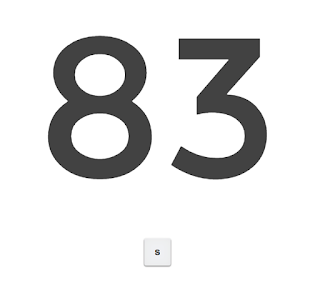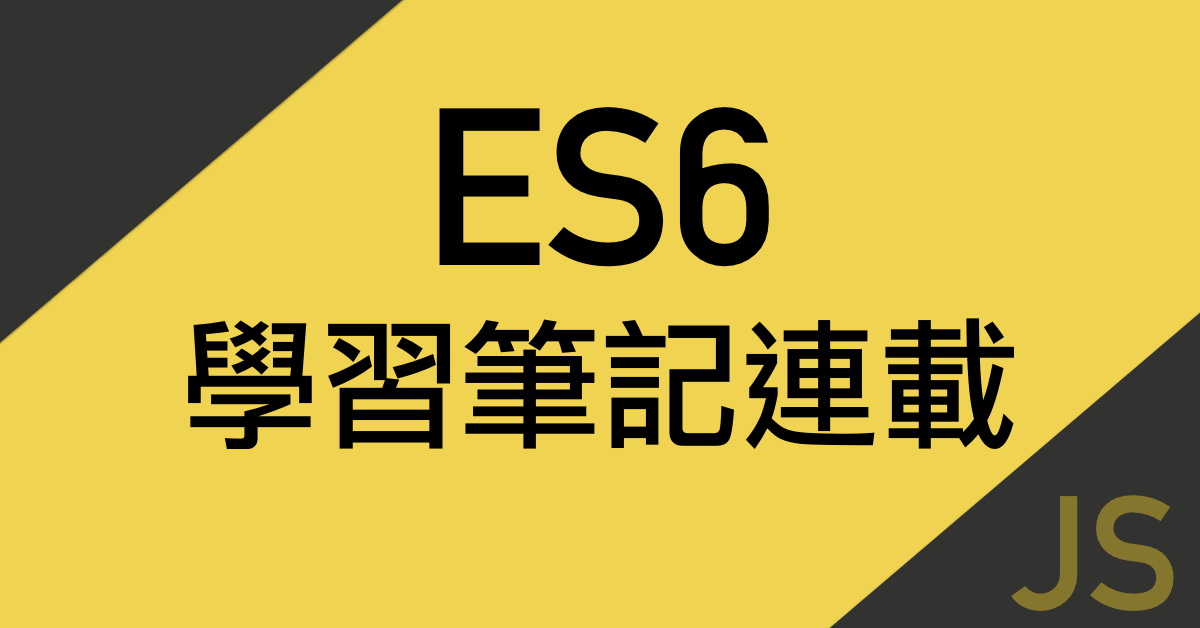# [筆記] JavaScript ES6 中的模版字符串（template literals）和標籤模版（tagged template）

### 模版字符串（template literal）的基本應用

``````
'<div class="banner">\n'+
'<img src="img1.jpg"\n'+
'</div>\n'+
```
```

``````
let component_es6 = `
<div class='banner'>
<img src="img1.jpg>
</div>
`
```
```

### 在模版字符串中嵌入變數

``````
let myName = "PJCHENder",
numA = 4,
numB = 7;

let content = `Hello, my name is \${myName}, my lucky number is \${2*(numA + numB)}`;

console.log(content);  // "Hello, my name is PJCHENder, my lucky number is 22"
```
```

``````
var greeting = `\`Hello\` World!`;

console.log(greeting); // "`Hello` World!"
```
```

### 進階：標籤模版（tagged template）

``````
console.log `Hello EveryOne`;    //  Array  ["Hello EveryOne"]
console.log('Hello EveryOne');   //  String "Hello EveryOne"
```
```

``````
let myName = "PJCHENder";
let myCountry = "Taiwan";

tag `<p> My name is \${myName} and my coutry is \${myCountry}</p>`;  //  使用標籤模版
tag(["<p> My name is ", " and my coutry is ", "</p>"], "PJCHENder", "Taiwan")  // 等同於上面這段
```
```

``````
let myName = "PJCHENder";
let myCountry = "Taiwan";

tag `<p> My name is \${myName} and my coutry is \${myCountry}</p>`;
// tag(["<p> My name is ", " and my coutry is ", "</p>"], "PJCHENder", "Taiwan")  // 等同於上面這一段

function tag(template){
console.log(template);
console.log(arguments);
}
```
```

``````
let myName = "PJCHENder";
let myCountry = "Taiwan";

tag `<p> My name is \${myName} and my coutry is \${myCountry}</p>`;
// tag(["<p> My name is ", " and my coutry is ", "</p>"], "PJCHENder", "Taiwan")  // 等同於上面這一段

function tag(template, ...values){
console.log(template);  //  ["<p> My name is ", " and my coutry is ", "</p>"]
console.log(values);  //  ["PJCHENder", "Taiwan"]
}
```
```截圖自：阮一峰-ECMAScript 6 入門

# [筆記] JavaScript ES6 中的 for ... of（處理陣列的好幫手）

### 陣列中 for...of 的基本用法

for...of 的使用非常簡單，以陣列為例：

``````
let arr = [10, 20, 30]

for(let value of arr){
console.log(value);  // 10, 20, 30
}
```
```

### for...of 的其它用法

for...of 除了用在陣列之外，也可以用在其他的資料型態，像是字串、map、set 等等...，舉字串為例：

``````
let string = "ES6";

for(let value of string){
console.log(value);  // "E", "S", "6"
}
```
```

# [筆記] JS30系列：監聽按鍵事件及撥放音效（Day1）

### 思考分析

1. 瀏覽器能夠辨認使用者在鍵盤上所按下的按鍵
2. 當鍵盤按下指定的按鍵後能夠觸發音效
3. 當鍵盤按下指定的按鍵後能夠觸發網頁上的元件產生相對應的變化
4. 網頁上元件的變化要能夠回覆成原本的狀態
5. 播放的音效要能夠停止

### 辨認使用者在鍵盤上所按下的按鍵

KeyCode：每一個按鍵其實都有對應的鍵碼（keycode），而我們可以透過使用者所按下的鍵碼來得知使用者是按了鍵盤上的哪一個按鈕。在 keycode.info 這個網站中，你就可以得到每一個按鍵所對應到的鍵碼。keycode @ keycode.info

``````
// 監聽鍵盤按鍵事件，並回傳所按的按鍵為何
console.log(e);
});
```
```

### 鍵盤按下指定按鈕後能夠觸發音效

data-* attribute：簡單說明一下什麼是 data-* attribute，有時我們會需要使用到某些自定義的屬性，但是為了要避免大家在 HTML 中隨意的添加屬性，於是在 HTML5 中就多了 data-* attribte 這個屬性，其中的 * 就是一個可以自定義的名稱，例如：data-key='83' 或者是 data-item='1'，關於 data-* attribute 屬性如果想要有更多的瞭解，可以參考（[教學] 什麼是 HTML 5 中的資料屬性）。

querySelector：這是 JS 中原生的 CSS 選擇器，裡面可以像 jQuery 一樣去選擇想要選擇到的元素，有一些要注意的地方像是，若找不到相對性的元素會回傳 null，否則會回傳第一個符合的元素，因此，若你是多個屬性具有相同的 class 名稱時，它只會選到第一個符合的元素。如果你是想要選擇多個具有相同 class 名稱的屬性時，就要用 querySelectorAll

``````
//  選擇鍵盤按鍵所對應到的網頁元素
const audio = document.querySelector(`audio[data-key="\${e.keyCode}"]`);
console.log(audio);
})
```
```

``````
//  播放元素音效檔
const audio = document.querySelector(`audio[data-key="\${e.keyCode}"]`);
if(!audio) return;  //  如果沒有按到對應的按鍵，則停止此函式
audio.play();       //  播放元素的音效
})
```
```

``````
const audio = document.querySelector(`audio[data-key="\${e.keyCode}"]`);
if(!audio) return;      //  如果沒有按到對應的按鍵，則停止此函式
audio.currentTime = 0;  //  讓每次回到音效檔的起始點
audio.play();           //  播放元素的音效
})
```
```

### 鍵盤按下指定按鈕後能夠網頁動畫效果

``````
//  監聽使用者按鍵事件，並取得對應的元素
const key = document.querySelector(`.key[data-key="\${e.keyCode}"]`);
})
```
```

classList 物件：接下來我們要做的就是讓按下按鍵之後，能夠在該元素上添加指定的 class 以產生動態的效果，可以利用 JS 中內建的 element.classList.add( ) 這個方法：

``````
const key = document.querySelector(`.key[data-key="\${e.keyCode}"]`);    //  取得按鍵對應的元素
})
```
```

transitionend event：這個 transitionend 事件主要是對應到 CSS 中 transition 的動畫效果，當這個 transition 效果執行結束的時候會引發事件。

querySelectorAll：為了監聽每一個按鍵的事件，這裡我們就會用到 querySelectorAll 這個方法，它會選出所有具有該 class 的元素，並回傳為一 NodeList

forEach( )：接著為了要重複的讀出這個 NodeList 的內容，我們會用到 forEach( ) 這個方法，forEach( ) 這個方法裡面可以放入 callback function，預設的參數包含該 NodeList 的屬性值和屬性名稱。因此我們可以寫出如下的語法：

``````
const keys = document.querySelectorAll('.key'); //  選出所有具有 .key 的元素，回傳成陣列
keys.forEach((key) => {key.addEventListener('transitionend', removeTransition)});   //  利用 forEach 方法讀取 NodeList 並為每一個元素加上 transitionend 事件，事件執行時會促發 removeTransition
```
```

``````
let removeTransition = function(e){
console.log(e);
}
```
```

``````
let removeTransition = function(e){
if(e.propertyName !== 'transform') return; //    省略掉其他 propertyName 不是 transform 的物件
this.classList.remove('playing');   //  把 playing 這個 class 移除
}
```
```

### 看一下我們學到了什麼

• 監聽事件（addEventListener(event, function)）--- 在 function 中代入參數會回傳和該事件有關的物件
• 按鍵事件（keydown
• 透過 CSS 選擇元素（querySelector, querySelectorAll)
• 音效相關（element.play( ), element.currentTime)
• 資料屬性（data-* attribute
• 動畫結束事件 --- transitionEnd
• forEach( )
• 若不符合則退出函式：function( ){if(...) return}

# [技術分享] 什麼是 HTML 5 中的資料屬性（data-* attribute）## HTML5 中的 data-* attribute 屬性

```<div id="slider" data-type="slideShow">
<img class='photo' data-item="1" data-size="xs" src="http://fakeimg.pl/350x200/?text=Hello" />
<img class='photo' data-item="2" data-size="lg" src="http://fakeimg.pl/550x200/?text=Welcome" />
</div>
```

## 使用 JS 取得 data-* attribute 的屬性值

```let slider = document.getElementById('slider');
console.log(slider.dataset.type);   //  "slideShow

let photo = document.querySelectorAll('.photo');
console.log(photo.dataset.item);   //  "1"
console.log(photo.dataset.size);   //  "xs"
```

```console.log(\$('#slider').data('type')); //  "slideShow"
```

## 透過 CSS 取得或選取 data-*attribute

```<article data-content="Hello Everyone">
</article>
```

```article::before{
content: attr(data-content);
}
```

```#slider img[data-size="xs"] {
width: 400px;
}
#slider img[data-size="lg"] {
width: 800px;
}
```

# [筆記] JS30 系列 Day0 - 課程說明

### 我聽到 JavaScript 就怕，這門課適合我嗎？

（1）先跳過，先抓住自己理解的部分，把成品做出來，因為如果你一直卡在不懂的地方，你可能會沒辦法享受這門課程。這就好像在讀英文文章的時候，如果你碰到每一個不懂的單字就停下來查字典，那麼你可能很難享受這篇文章（有許是文章太難了，也許可以先試著讀懂文章的大意就好）。

# [筆記] JavaScript ES6 中的展開運算子（spread operator）和其餘運算子（rest operator）

### 其餘運算子（rest operator）

``````
let arr = [1,2,3,4,5];

let avg = function(arr){
let sum = 0;
for(let i = 0; i < arr.length; i++){
sum += arr[i];
}
return sum / arr.length;
}

console.log(avg(arr));  //  3
```
```

``````
let avg = function(arr){
let sum = 0;
for(let i = 0; i < arr.length; i++){
sum += arr[i];
}
return sum / arr.length;
}

console.log(avg(1,3,5,7,9));  // NaN
```
```

``````
let avg = function(...arr){
console.log(arr)  // [1,3,5,7,9]
let sum = 0;
for(let i = 0; i < arr.length; i++){
sum += arr[i];
}
return sum / arr.length;
}

console.log(avg(1,3,5,7,9));  // 5
```
```

``````
let number = [1,2,3,4,5];

console.log(Math.max(number));  //  NaN
```
```

``````
let number = [1,2,3,4,5];

console.log(Math.max(...number));  //  5

console.log(...number);  // 1,2,3,4,5
```
```

# [筆記] 親手打造屬於你自己的 JavaScript Framework/Library（下）

### HTML部分

``````
<body>
<div id="app">
<select id='Lang'>
<option value="en" selected>English</option>
<option value="zh">中文</option>
</select>
<button>登入</button>
</div>
<h1 id="logMsg"></h1>
</div>

<script src="jquery-3.1.1.js"></script>
<script src="greetr.js"></script>
<script src="app.js"></script>
</body>
```
```

### JS 部分（greetr.js）

``````
greetHTML: function(selector, formal) {
if (!\$) throw "jQuery not loaded";

if (!selector) throw "no seletor";

let msg = "";

if (formal) {
msg = this.formalGreeting();
} else {
msg = this.greeting();
};

\$(selector).html(msg);

return this;

}
```
```

```;
(function(global, \$) {

var Greetr = function(firstname, lastname, language) {
return new Greetr.init(firstname, lastname, language);
}

let supportedLangs = ['en', 'zh'];

let greetings = {
en: 'Hello',
zh: '你好'
};

let formalGreetings = {
en: 'Greetings',
zh: '您好'
};

let logMessages = {
en: 'Logged',
zh: '登入'
};

Greetr.prototype = {
fullName: function() {
return this.firstname + ' ' + this.lastname;
},
validate: function() {
if (supportedLangs.indexOf(this.language) === -1) {
throw 'Invalid Language';
}
},
greeting: function() {
return greetings[this.language] + ' ' + this.firstname;
},
formalGreeting: function() {
return formalGreetings[this.language] + ', ' + this.fullName();
},
greet: function(formal) {

let msg;

// if undefined or null, it will coerced to 'false'
if (formal) {
msg = this.formalGreeting();
} else {
msg = this.greeting();
}

if (console) {
console.log(msg);
}

// 'this' refers to the calling object at execution time
// makes method chainable
return this;

},
log: function() {
if (console) {
console.log(logMessages[this.language] + ' ' + this.fullName());
}
return this;
},
setLang: function(lang) {
this.language = lang;
this.validate();
return this;
},
greetHTML: function(selector, formal) {
if (!\$) throw "jQuery not loaded";

if (!selector) throw "no seletor";

let msg = "";

if (formal) {
msg = this.formalGreeting();
} else {
msg = this.greeting();
};

\$(selector).html(msg);

return this;

}
};

Greetr.init = function(firstname, lastname, language) {
let self = this;
self.firstname = firstname || '';
self.lastname = lastname || '';
self.language = language || 'zh';
self.validate();
}

Greetr.init.prototype = Greetr.prototype;

global.Greetr = global.G\$ = Greetr;

}(window, jQuery))
```

### Library 應用（app.js）

``````
let pj = G\$("Po-Jung", "Chen");
pj.setLang(\$("#Lang").val()).greetHTML('#logMsg', true);
})
```
```

→回到此系列文章目錄

# [筆記] JavaScript ES6 中的物件的擴展（object literal extension）

### 物件的擴展（object literal extension）

``````
let website = "pjchender";
let obj = {website};
console.log(obj);    //[Object]{website: "pjchender"}
```
```

``````
let website = "pjchender";
let obj = {abc:website};
console.log(obj); // [object]{abc: "pjchender}
```
```

``````
let website = "pjchender";
let country = "Taiwan";

let obj = {
website,
country
};
console.log(obj); // [object]{country: "Taiwan", website: "pjchender"}
```
```

``````
let name = "PJCHEN";
let country = "Taiwan";

let obj_es6 = {
name: "Aaron",
country,
}

console.log(obj_es6); // [Object]{name: "Aaron", country: "Taiwan"}
```
```

### 物件中的方法也可以簡寫

``````
let name = "PJCHEN";
let country = "Taiwan";

let obj = {
name,
country,
location(){
console.log(this.name + ' lives in ' + this.country);
}
}

obj.location();  // PJCHEN lives in Taiwan
```
```

``````
let obj = {
name: name,
country: country,
location: function(){
console.log(this.name + ' lives in ' + this.country);
}
}
```
```

### 在 ES6 中允許將表達式作為屬性名稱，以達到動態賦值的效果

``````
let obj_es = {
["web"+"site"]: "pjchender"
}
console.log(obj_es); // [Object]{website: "pjchender"}
```
```

``````

let websiteName = "pjchender";
let a = 2;
let b = 3;

let obj_es = {
[websiteName]: "welcome",
[a+b]: "sumNumber"
}
console.log(obj_es);  // [Object]{5: "sumNumber", pjchender: "welcome"}

```
```

# [筆記] JavaScript ES6 中的物件解構賦值（object destructuring）

### 物件解構賦值的基本使用

``````
let obj = {
website: "pjchender",
country: "Taiwan"
}

let {website, country} = obj;
console.log(website);  // pjchender
console.log(country);  // Taiwan
```
```

``````
let {website:website, country:country} = obj;
```
```

``````
let obj = {
website: "pjchender",
country: "Taiwan"
}

let {website:wb, country:ct} = obj;

console.log(website, country);   //  Error:website in not defined
console.log(wb, ct)  // "pjchender", "Taiwan"

```
```

``````
// website 和 wb 並沒有相對應
let{website} = {wb: "pjchender"};
console.log(website);    // undefined
```
```

### 物件解構賦值是以屬性名稱做對應

``````
let [a, , c] = [1, 2, 3];
console.log(a, c); // 1, 3
```
```

``````
let{a, ,c} = {a:1, b:2, c:3};
console.log(a,c); // Error
```
```

### 物件解構賦值同樣能給予預設值此範例擷取自阮一峰-ECMAScript 6 入門

### 物件解構賦值的用途

``````
let data_JSON = {
id: 74,
website: "pjchender",
country: "Taiwan",
detail:{
phone: "0933333333"
}
}

let {id, website, country, detail} = data_JSON;
console.log(id, website, country, detail);
```
```

# [筆記] JavaScript ES6 中的陣列解構賦值（array destructuring）## 陣列解構賦值的方法（array destructuring）

``````let numbers = [1, 2, 3];
let a = numbers;
let b = numbers;
let c = numbers;
console.log(a,b,c);   // 1, 2, 3
``````

### 一般用法

``````let [a, b, c] = [1, 2, 3];
console.log(a, b, c); // 1, 2, 3
``````

### 當輸入的變數多於所給的值

``````// 當變數多於所給的值
let [a, b, c, d] = [1, 2, 3];
console.log(a, b, c, d); // 1, 2, 3, undefined
``````

### 當輸入的變數少於所給的值

``````// 當變數少於給的值
let [g, , i] = [1,2,3];
console.log(g, i);  // 1, 3
``````

### 在陣列解構中賦予預設值

``````let [a, b, c = 4, d = 'Hello'] = [1, 2, 3];
console.log(a, b, c, d); // 1, 2, 3, "Hello"
``````

## 防雷須知

``````let x = 0
let y = 0
console.log(x, y)
[x, y] = [1, 2]
console.log(x, y)
``````

# [筆記] JavaScript ES6 中的函數預設值（default value）

### 函式預設值的使用

``````
function add(x = 3, y = 5){
console.log(x+y);
}

```
```

### 使用預設值的基本觀念

``````
console.log(x+y);
}

```
```

``````
console.log(x+y);
}

```
```

# [筆記] JavaScript ES6 中的箭頭函數（arrow function）及對 this 的影響

### 什麼是箭頭函數（arrow function）### 箭頭函數當中的 this 是定義時的對象，而不是使用時的對象

``````
// 原本的 function
let fn = function(){
console.log(this.constructor.name);  // Object(data)
setTimeout(function(){
console.log(this.constructor.name) // Window
},100);
}

// 箭頭函式 Arrow function
let fn_arr = function(){
console.log(this.constructor.name);  // Object(data)
setTimeout(() => {
console.log(this.constructor.name) // Object(data)
},100);
}

let id = 21;
let data = {
id: 21
}

fn.call(data);
fn_arr.call(data);
```
```

setTimeout：裡面都分別帶入 setTimeout 的函式，我們知道 setTimeout 執行的時間會在整個 JS execution context 都被執行完後才會執行（如果對這概念不清楚的話可參考：[筆記] 談談JavaScript中的asynchronous和event queue），因此函式建立的時間和實際執行的時間是不同的，因此這也創造了兩個不同時間點的 this 所指稱的對象。

call：另一個要了解的是 call 的用法，在 call 當中，我們會帶入後面所指定的物件當作所指稱的 this 對象（對這個概念不清楚的話可參考：[筆記] 了解function borrowing和function currying ─ bind(), call(), apply() 的應用）。

this.constructor.name：這樣子的寫法只是避免在回傳出物件的時候把整個物件內容給傳出來，而是指示傳出該物件的名稱（參考自：stackoverflow）。

``````
var button = document.querySelector('button');
var fn_arr = () => {
// 建立 function 時 this 指 Window
console.log(this.constructor.name)  // 執行function時 this 指 Window
};
var fn = function(){
// 建立 function 時 this 指 Window
console.log(this.constructor.name)  // 執行function時 this 指 HTMLButtonElement

```
```